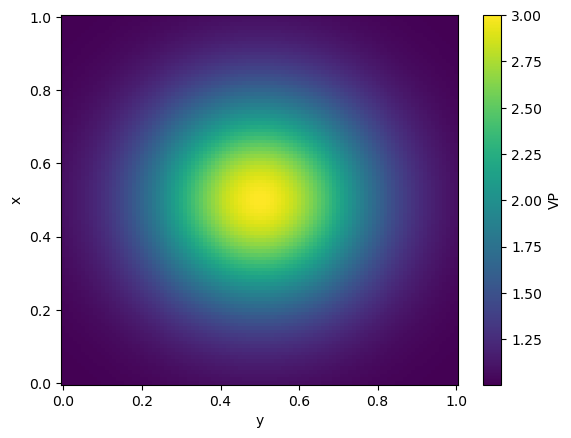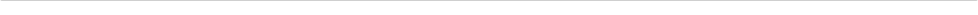Version:This tutorial is presented as Python code running inside a Jupyter Notebook, the recommended way to use Salvus. To run it yourself you can copy/type each individual cell or directly download the full notebook, including all required files.Copy
%matplotlib inline

import numpy as np
import xarray as xr
import salvus.namespace as sn
from salvus.mesh.unstructured_mesh_utils import extract_model_to_regular_grid

# Working with variable models and extracting slices

## Micro tutorial

In this tutorial we'll make a few simple meshes, and see how we can extract the model parameters on those meshes for visualization and analysis. This will allow us to visualize the meshed models along different depth slices, as well as convert them to a regularly-sampled representation that may be easier to share with others.
Salvus uses xarray to handle models defined on regular grid. This allows for a simple way to interpolate models onto unstructured grids. We recommend reading xarray's documentation for a deeper understanding how its indexing, plotting, and interpolation functions work.
On the Salvus side, the extraction algorithm consists of two critical steps:
1. The elements enclosing each point are found, and
2. The material parameters are interpolated to each point using the mesh's GLL basis.
Since the mesh may be fully unstructured, the enclosing element cannot be found via a simple coordinate lookup, and as such the runtime of the extraction routine scales with the size of the inputs. For many cases (>10k elements, >100k points), however, a runtime of several seconds can be expected. A progress bar is available by passing verbose=True to the extract_model_to_regular_grid function.
Points passed which are outside of the mesh are labeled with np.nan, following the standard conventions for missing data. Note that having many points outside of the mesh may negatively affect the enclosing-element algorithm, so it is good to keep these more-or-less in line with the dimensions of your domain. This is not always the case when topography is present. In these cases you may see a message reporting "N points were not claimed by enclosing elements". You may want to adjust the extraction points to keep these at a a minimum, but with deformed meshes or those not aligned with coordinate axes some points outside the mesh are inevitable, and the message need not be a concern.
Each section here -- 2-D, 3-D, and 3-D Spherical -- can be understood independently of the others.

# 2-D

## Build model

# Gaussian
x, y = np.linspace(0.0, 1.0, 101), np.linspace(0.0, 1.0, 101)
xx, yy = np.meshgrid(x, y, indexing="xy")
g = np.exp(-(((xx - 0.5) ** 2 + (yy - 0.5) ** 2) / (2 * 0.2**2)))

# Pars
vp = 2 * g + 1
rho = vp / 2

# Xarray dataset
ds_model_2d = xr.Dataset(
coords={"x": x, "y": y},
data_vars={"VP": (["x", "y"], vp), "RHO": (["x", "y"], rho)},
)

# Salvus wrapper.
m = sn.model.volume.cartesian.GenericModel(name="blob", data=ds_model_2d)

# Plot
m.ds.VP.plot()
<matplotlib.collections.QuadMesh at 0x7f23ebe11710>## Initialize project

p_2d = sn.Project.from_volume_model("proj_2d", m, True)## Define a simulation

The simulation configuration here will also be used for the 3-D example.
sc = sn.SimulationConfiguration(
name="blob_fmax",
event_configuration=None,
elements_per_wavelength=2.0,
max_frequency_in_hertz=10.0,
model_configuration=sn.ModelConfiguration(
background_model=None, volume_models="blob"
),
)

p_2d.add_to_project(sc, overwrite=True)

## Get and visualize simulation mesh

mesh_0 = p_2d.simulations.get_mesh("blob_fmax")
mesh_0
[2023-09-10 18:16:37,135] INFO: Creating mesh. Hang on.